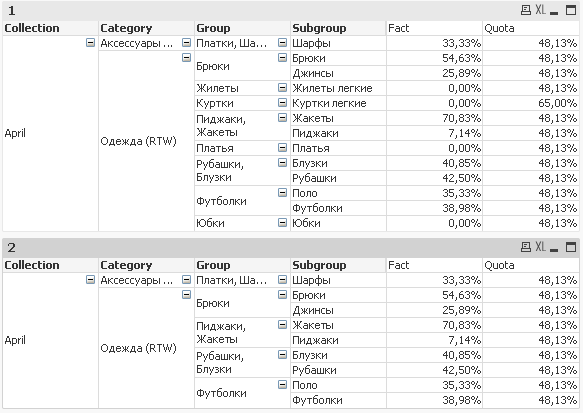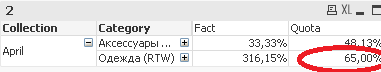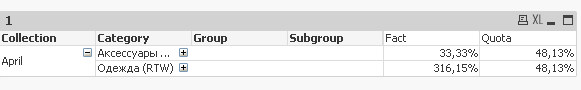QlikView App Development

Discussion Board for collaboration related to QlikView App Development.

Highlighted
Contributor II

Calculate Quota expression by Fact values in Pivot Table

Hi frends !

I faced the next problem. I need to show Quota on by Fact values in Pivot Table on the all levels of aggregations. How I can do that?

On the screenshot below chart "1" Quota and Fact expressions, but if we hide Quota expression, in Fact there will be no zeros.

In chart "2" - look's like result what we need, BUT it works on the 4 and 3 level of Dimentionality(), because we wrote, the next expression:

```If("Fact">0,
Max( TOTAL<Collection, Category, Group, Subgroup>
Aggr(
Sum (Quota)
, Collection, Category, Group, Subgroup)
)
)

```

and it dont works when we looking at "Quota" on Category and Collection level.Error looks like here:Because expression we wrote to Quota stops working when the Fact > 0, and when we drill down to the Collection and Category, Fact still > 0.

So hope that clearly explaned the current problem.

May be we need to use the calculated dimention, but how i'll be aggregating Quota expression by calculated dimention?

Final result shoul look like here:andAnother words we need to say to the Quota expression: Look at the last granular level, find only Fact > 0 and show Quota only by those fields (values of fields), where Fact > 0.

P.S.: Demo source in attachment.

Tags (5)
1 Solution

Accepted SolutionsNot applicable

Re: Calculate Quota expression by Fact values in Pivot Table

IF "Sum(Fact)" have big Formula with "AGGR" and other functions, method by sunindia don`t work.

This method will work with big formulas.

1) we create "Variable" For string with only true Subgroup(last DIM in AGGR functions)

vTest = CHR(39)&CONCAT(IF( Aggr(Sum (Fact) , Collection, Category, Group, Subgroup) >0, Subgroup),CHR(39)&','&CHR(39))&CHR(39)

2) Add to "set analysis" in "Sum (Quota)". => Sum ({<Subgroup={\$(vTest)}>} Quota)

Now in set analysis, we will take only Subgroups where "Sum (Fact)>0".

5 RepliesMVP

Re: Calculate Quota expression by Fact values in Pivot Table

Try this expression:

Max(TOTAL <Collection, Category, Group, Subgroup> Aggr(If(Sum(Fact) > 0, Sum(Quota)), Collection, Category, Group, Subgroup))MVP

Re: Calculate Quota expression by Fact values in Pivot Table

Oh so you still want to see the 0's when you have the Group and Subgroup Expanded???

Is that the goal?MVP

Re: Calculate Quota expression by Fact values in Pivot Table

What if you use Min instead of Max?

Min(TOTAL <Collection, Category, Group, Subgroup> Aggr(Sum(Quota), Collection, Category, Group, Subgroup))Not applicable

Re: Calculate Quota expression by Fact values in Pivot Table

IF "Sum(Fact)" have big Formula with "AGGR" and other functions, method by sunindia don`t work.

This method will work with big formulas.

1) we create "Variable" For string with only true Subgroup(last DIM in AGGR functions)

vTest = CHR(39)&CONCAT(IF( Aggr(Sum (Fact) , Collection, Category, Group, Subgroup) >0, Subgroup),CHR(39)&','&CHR(39))&CHR(39)

2) Add to "set analysis" in "Sum (Quota)". => Sum ({<Subgroup={\$(vTest)}>} Quota)

Now in set analysis, we will take only Subgroups where "Sum (Fact)>0".

Contributor II

Re: Calculate Quota expression by Fact values in Pivot Table

I'm using complex formula for Fact with Aggr func, then aggr(sum(aggr(sum...doesn't works...

But the final solution - is to use peace of SunIndia logic "If(Sum(Fact) > 0" and Max logic with variable "CHR(39)&CONCAT(IF( Aggr(Sum (Fact) , Collection, Category, Group, Subgroup) >0, Subgroup),CHR(39)&','&CHR(39))&CHR(39)" to.

Thanks a lot for yous help.